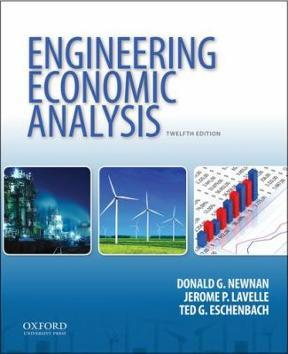×
×

# First Bank is sending alumni of universities anISBN: 9780199339273 93

## Solution for problem 3-50 Chapter 3

Engineering Economic Analysis | 12th Edition

• Textbook Solutions
• 2901 Step-by-step solutions solved by professors and subject experts
• Get 24/7 help from StudySoup virtual teaching assistantsEngineering Economic Analysis | 12th Edition

4 5 1 355 Reviews
15
2
Problem 3-50

First Bank is sending alumni of universities an invi-tation to obtain a credit card, with the name of theiruniversity written on it, for a nominal 9.9% interestper year after 6 months of 0% interest. These inter-est rates apply to the outstanding debt if not paid bya specified date each month, and hence interest iscompounded monthly. If you fail to make the mini-mum payment in any month, your interest rate couldincrease (without notice) to a nominal 19.99% peryear. Calculate the effective annual interest rates thecredit company is charging in both cases

Step-by-Step Solution:
Step 1 of 3

Math 113 October 29, 2018 The formula for Simple Interest is i=prt, where i stands for interest, p stands for the principal amount, r stands for the annual interest rate, and t stands for time. Say you lend \$1,000 at 4% annual for one year. How much interest did you make Well, we are solving for i, so we simply plug in the rest of the problem into the equation....

Step 2 of 3

Step 3 of 3

##### ISBN: 9780199339273

Unlock Textbook Solution